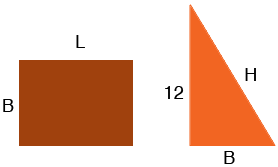SEARCH HOMEMath Central Quandaries & QueriesQuestion from rajesh, a student: The perimeter of a rectangle is equal to the perimeter of a right-angled triangle of height 12 cm if the base of the triangle is equal to the breadth of the rectangle, what is the length of the rectangle?Rajesh,

There is not enough information to solve this problem.

I let the length of the rectangle be $L$ cm, its breadth be $B$ cm and the hypotenuse of the triangle be $H$ cm.You want $2L + 2B = 12 + B + H.$ Since the triangle is a right triangle, from Pythagoras' Theorem you know that $12^2 + B^2 = H^2 .$

To illustrate that there is not enough information let $B = 5$ cm. Then using Pythagoras' Theorem $H = 13$ cm and the perimeter of the triangle is $12 + B + H = 30$ cm. Since the perimeter of the rectangle is also 30 cm you must have $L = 10$ cm.

Now suppose $B = 7$ cm then Pythagoras' Theorem gives $H = 13.89$ cm which means $L = 9.45$ cm.

Now you try one, say $B = 15$ cm. What do you get for $L?$

There are many possible values for $L.$ Is there something missing in your wording of the problem?

PennyMath Central is supported by the University of Regina and the Imperial Oil Foundation.